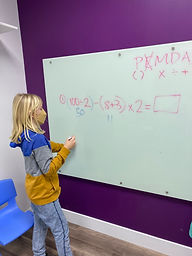Ms. Briana

Target 1​

Lesson Type:

Continuation

Number Operation

:

Computation

Solve multi-step problems within 100 (addition, subtraction, multiplication, and division).

1:

Solve problems that have more than one operation.

2:

Understand that the presence of parenthesis in an arithmetic equation signals for this part of the problem to be solved first.

3:

Understand when solving a problem that has both addition and subtraction operations that solving starts on the left and continues to the right.

4th

Vocabulary:

Order of Operations: The order in which multi-step equations are solved.

Activities:

Students threw bean bags at the at the whitr board to pick which multi step equation to solve. Students then used the answers to the equations to make off numbers on their bingo cards.

Students worked together to figure out multi-step equations by using the order of operations.Home Exploration

Guiding Questions:Absent Students:

Elijah

Target 2

:

Vocabulary:

Activities:Home Exploration

Guiding Questions:Target 3

:

Vocabulary:

Activities:Home Exploration